Research Summary- Jesús Antonio De Loera.

I started my career working in convex & discrete geometry, and computational geometry. My work in the combinatorics and geometry of convex polytopes is well-known. In my dissertation, I solved an open question of mathematicians Gelfand, Kapranov and Zelevinsky by finding an example of a non-regular triangulation of the cartesian product of two simplices . Triangulations appear everywhere, even in the context of Groebner bases of toric ideals. Later I worked very deeply on the problem of finding optimal triangulations and subdivisions of polyhedra. For example in joint work with Below and Richter-Gebert we proved that it is NP-hard to find the smallest triangulation for a 3-dimensional polytope, but I also work on practical ways to find those minimal triangulations for concrete instances (e.g., the minimum triangulation of the dodecahedron has 23 tetrahedra, shown in my main web site). My first book joint with Joerg Rambau and Francisco Santos Triangulations: Structures for Algorithms and Applications was published by Springer in 2010. It is a thorough reference about triangulations of polyhedra.

I have also published many noteworthy contributions to the problems of computing volumes and integrals of polyhedra , and about counting lattice points. The list of applications of these computational problems is very large; from algebraic geometry and representation theory (toric varieties, Gelfand-Tsetlin and Clebsch-Gordan coefficients) to combinatorics (e.g., matroids), computer algebra, and probability and statistics . I have also contributed widely to understanding Ehrhart polynomials, in both theory and practice. For example, I have enjoyed looking at their roots and in joint work with Liu and Yoshida we found the first ever closed-form combinatorial formula for the volumes and Ehrhart coefficients of the Birkhoff polytope, i.e., the convex hull of allpermutation matrices. Our well-known software LattE was started under my direction and initiative, used for research by many mathematicians, and introducing dozens of undergraduates to research. In the current version it can compute Ehrhart quasipolynomials, integrals and volumes.

In the past seven years, I have applied algebra and geometry in the area of integer and combinatorial optimization. This the part of applied mathematics related to optimizing functions over discrete sets. A famous example of such problem is the traveling salesman problem: Given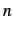cities that must be visited only once, and the costsof flying from city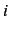to city, the goal is to find the best order to visit all the given cities in order to minimize the total cost of the trip.

I have been one of the leaders of a new approach to the theory of optimization. In this new point of view, tools from algebra, topology and geometry, that were considered too pure and unrelated to applications, are used to prove unexpected computational or structural results. My new second book (joint with Raymond Hemmecke and Matthias Köppe) Algebraic and Geometric Ideas in the theory of Discrete Optimization is the first compilation of results from this novel approach collecting the work of several mathematicians. My own work has provided fundamental new developments taking place in the area of discrete optimization which I summarize here:

1. Traditionally discrete optimization has focused on how to solve problems with only linear equations or constraints. In pioneering work published in 2006, joint with Hemmecke, Köppe and Weismantel) we proved the first theoretical results for the more difficult model with non-linear objective functions. To put them in context, recall the famous result obtained by H.W. Lenstra Jr. in 1983, states that when the number of variables is fixed and both the objective function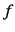and constraintsare linear polynomials, then there is an algorithm to solve it in polynomial time on the input size. Similarly, in another breakthrough, in 2002, L. Khachiyan and L.Porkolab proved that the same holds when the number of variables is fixed and the objectiveis a convex polynomial. It is thus a natural question to ask: if we continue to fix the number of variables and theare linear, but the objective functionis an arbitrary non-linear polynomial, what is the computational complexity?

We extended the Lenstra's and Khachiyan's results, we answered the question in a paper appeared in papers Mathematics of Operations Research and Math. Programming : We proved that while the problem is NP-hard, even for fixed dimension 2 and degree 4 polynomials, there is a new approximation algorithm to maximize an arbitrary integral polynomial over the lattice points of a convex rational polytope with a fixed dimension. The algorithm produces a sequence of upper and lower bounds for the global optimal value. Surprisingly this is an excellent approximation scheme. The new method codifies the (exponentially many) lattice point solutions, as a short (polynomial-size) multivariate rational generating function (sum of quotients of polynomials) based on the theory put forward by A. Barvinok (we did in LattE the first ever implementation of Barvinok's algorithm). Later Hemmecke, Köppe and I showed that gives a similar strong result for integer multiobjective optimization.

2. An integer linear program (ILP) is the problem of finding, for given matrixand vectors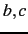, the minimum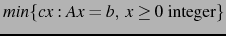. The problem is well-known to be NP-hard; thus one does not expect that a general efficient algorithm can be found. For an ILPa test set is a finite set of integral vectors such that every feasible non-optimal solution can be improved by adding a vector from the test set. The latticehas a natural partial order. Forwe say thatis conformal to, denoted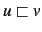, if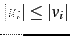and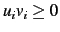for, that is,andlie in the same orthant ofand each component ofis bounded by the corresponding component ofin absolute value. The Graver basis of an integer matrixis the set of conformal-minimal nonzero integer dependencies on. For example, ifthen its Graver basis is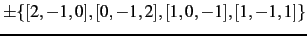.

Graver bases are in fact connected to commutative algebra and algebraic geometry, they are a universal Gröbner bases for the toric ideal associated to the matrix. During the past fifteen years, algebraic geometry and commutative algebra tools have showed exciting potential to solve problems in integer optimization. But, in the past, algebraic methods had always been considered guilty'' of bad computational complexity, namely, the notorious bad complexity for computing general Gröbner bases when the number of variables grow. Our research demonstrated that, by carefully using the structure of matrix, Graver bases can compete (and win!!!) against mainstream tools in optimization. The paper that initiated this idea appeared in the journal Discrete Optimization:

Fix any pair of integer matricesand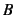with the same number of columns, of dimensions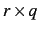and, respectively. The n-fold matrix of the ordered pairis the followingmatrix,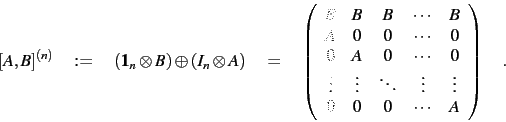The number of variables grow, but we can prove

Theorem Fix any integer matricesof sizesand, respectively. Then there is a polynomial time algorithm that, given anyand any integer vectorsand, solves the corresponding n-fold integer programming problem.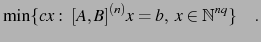The key ingredient to make it work comes from commutative algebra. What happens is that for every pair of integer matricesand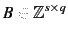, there exists a constant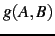such that for all, the Graver basis ofconsists of vectors with at mostthe number nonzero components. This means that it suffices to compute the Graver bases of a small-fold matrix to be able to obtain others. More recently the above theorem has been extended to optimization of convex functions instead of just optimizing linear functions.

3. An important family of integer linear programs are the multi-index transportation problems. These polytopes consist of all real-valued tables, arrays whose entries satisfies given sums from the entries (e.g., row sums, column sums equal to some values). Transportation polytopes, their integer points (called contingency tables by statisticians), and their projections, have been used and appear extensively in the operations research literature due to their importance on scheduling and planning. Their name was coined by Nobel-prize economist T. Koopmans who was interested on the efficient transportation of good by ships.

Back in 1986 several open problems were proposed by Vlach and co-authors. 20 year later, in our paper in SIAM Journal of Optimization , S. Onn and I solved most of them as easy corollaries of the following theorem

Theorem: (De Loera-Onn) Any integer programming problem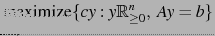is in fact polynomial-time representable as a-way transportation problem with-marginalsdepending polynomially on the binary size of the input:By saying that an integer program is representable as another we mean that the solution spaces are identical and one can transfer one into the other efficiently. Thus, one can interpret our theorem as a normal form'' or canonical reformulation'' algorithm. So, every integer or linear programming problem can be put in the special form of a multiway transportation problems for arrays of 3 indices with line sums prescribed.

4. For applications I have been interested on new methods to solve structured systems of polynomial equations and/or inequalities arising in discrete mathematics and optimization. Recently we worked on on a new technique that reduces non-linear polynomial systems of equations to the solvability of a sequence of large (but sparse) systems of linear equations over finite fields. In 2010 I received the INFORMS computer society award (ICS prize). The ICS Prize is an annual award for best English language paper or group of related papers dealing with the Operations Research/Computer Science interface. INFORMS (The Institute for Operations Research and the Management Sciences) is the largest international organization dedicated to operations research and the science of management. Here is what the prize citation says about the winning papers in combinatorics probability and computing, and in journal of symbolic computation

These two papers introduce a pioneering new approach for proving the optimality of solutions to combinatorial optimization problems. The approach begins by encoding the instance and an objective bound as a system of polynomial equations over a finite field. Then, using Hilbert's Nullstellensatz, the authors demonstrate the effectiveness of a computational method to certify the infeasibility of the polynomial system. The encoding is derived and analyzed for a number of problem classes, such as the k-coloring, stable set, longest cycle, largest planar subgraph, and minimum edge coloring problems. While the degree of the certifying polynomial could be exponentially large, the authors demonstrate that in many cases of interest the degree is low enough to allow for explicit, fast computations. The authors also develop a variety of computational enhancements, including computing over finite fields, exploiting symmetry, adding redundant equations, and applying alternative Nullstellensätze that allow them to solve 3-coloring problems significantly faster than existing methods.

In this impressive work, the authors take up a mathematical machinery that seemed very unlikely to be useful in practice and turn it into a useful computational algorithm. This work is likely to stimulate additional research into computational polynomial methods, perhaps placing them on the same footing as polyhedral techniques for solving combinatorial optimization problems.''

5. Most recently, I have studied the complexity and geometry of various linear optimization algorithms, most notably, the simplex method and interior point methods. The central path is the key object in interior point methods algorithms and software. In joint work with Vinzant and Sturmfels (paper published in Foundations of Computational Math, we gave exact algebraic formulas for the central path, determined the degree, and genus of the curve. These invariants, along with the degree of the Gauss image of the curve, are expressed in terms of a matroid of the input data. As an application we give an instance-specific bound of the total curvature of the central path, a quantity relevant for the performance of interior point methods. The key mathematical liaison'' we managed to established is that the differential geometry of a central curve is intimately connected to that of the underlying matroid of the constraints.

Another significant contribution regards the geometry of the simplex method. It is of course a long-standing open question to bound the diameter of the graphs of convex polyhedra. It is well-known in linear optimization that bounding the diameter is important for the performance of the simplex, which searches the graph of the polyhedron. Fields medalist Steven Smale has listed the problem of finding a strongly polynomial algorithm for linear programming, possibly via a polynomial pivot rule for the simplex method, as one of the key mathematical problems in the 21st century. Billera and Provan had proposed in 1979 a conjecture that if true would have proved a linear bound to the diameter of all polyhedra, namely twice the number of facet defining inequalities. The idea relies on a clever decomposition of simplicial complexes by a sequence of removals of lower dimensional faces. In a very recent paper published in Mathematics of Operation Research in 2012 (joint work with former postdoc Steve Klee), we solve their open question about their method to bound the diameter of convex polyhedra on the negative. We disproved the conjecture showing that the conjecture fails in a 4-dimensional example coming from transportation optimization problems. The construction is very elegant and uses topological tools (e.g., simplicial complexes deletion and contraction of edges).